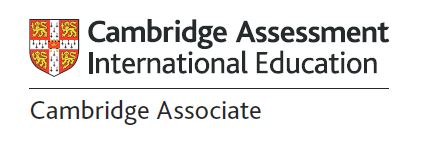School News
Posted on 08 May, 2019

# Maths Focus- 3 Plevko

In 3 Plevko we are learning to add two numbers using different strategies. We have been trying out each strategy to see which one we like best. We are using mental maths to help us. Mental maths is the process of finding an exact answer to a problem ‘in your head’. Mental maths is also used to find an estimated answer. To use mental maths, sometimes, we need to use abilities like decomposing numbers, or breaking apart numbers, to achieve our answers.

Add 10s to a 2 digit number by skip counting:

We practiced counting by tens from different starting points. This helped us to add multiples of 10 to another 2 digit number by skip counting on. For example 45+30=? Using this strategy, you would count by 10s three times. 55, 65, 75 so 45+30=75.

Add the tens and then the ones:

For two 2-digit numbers, we tried breaking apart the numbers into tens and ones adding them together and then putting it all together to find an answer. For example 42+31= 40+30+2+1=73

Move only the tens across:

For this strategy you can add two 2-digit numbers by moving only the tens across and then finally adding the ones. For example 42+33= 72+3=75. This is an extension of our first strategy, where we count by 10s from 42, and then add the ones afterwards.

## School hours: Monday - Friday

Primary School: 08:15 AM - 2:45 PM
Supervision is provided before school (8:00 AM - 8:15 AM)
and after school (2:45 PM - 3:00 PM).

Preschool: 08:00 AM - 2:00 PM

Front Office: 08:00 AM - 3:30 PM

Your independent public school right in the heart of Darwin